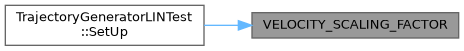moveit2 The MoveIt Motion Planning Framework for ROS 2.
unittest_trajectory_generator_lin.cpp File Reference
`#include <memory>`
`#include <gtest/gtest.h>`
`#include <pilz_industrial_motion_planner/joint_limits_aggregator.h>`
`#include <pilz_industrial_motion_planner/trajectory_generator_lin.h>`
`#include <pilz_industrial_motion_planner_testutils/command_types_typedef.h>`
`#include <pilz_industrial_motion_planner_testutils/xml_testdata_loader.h>`
`#include "test_utils.h"`
`#include <moveit/kinematic_constraints/utils.h>`
`#include <moveit/robot_model/robot_model.h>`
`#include <moveit/robot_model_loader/robot_model_loader.h>`
`#include <moveit/robot_state/conversions.h>`
`#include <moveit/robot_state/robot_state.h>`
`#include <rclcpp/rclcpp.hpp>`
Include dependency graph for unittest_trajectory_generator_lin.cpp: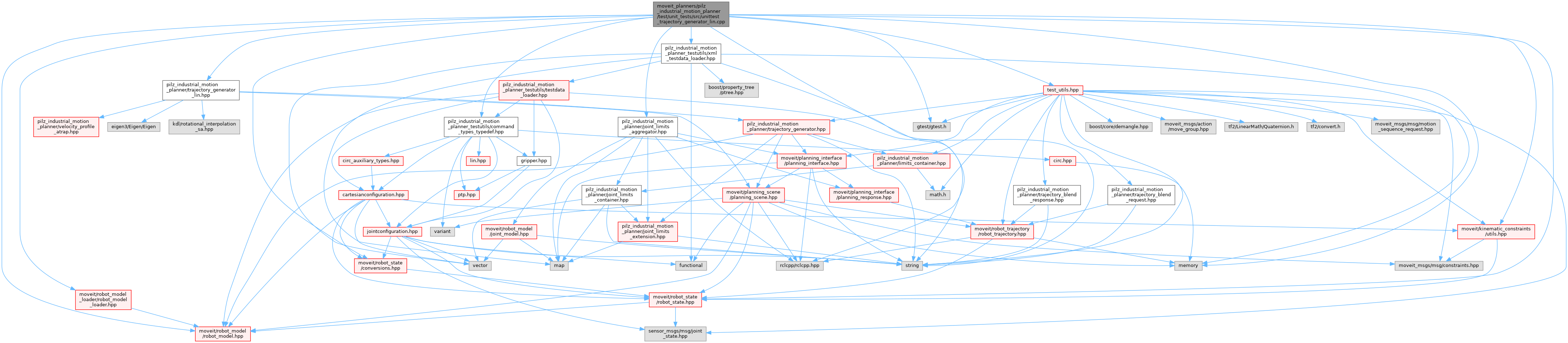Go to the source code of this file.

## Classes

class  TrajectoryGeneratorLINTest
Parameterized unittest of trajectory generator LIN to enable tests against different robot models.The parameter is the name of robot model parameter on the ros parameter server. More...

## Functions

const std::string TEST_DATA_FILE_NAME ("testdata_file_name")

const std::string PARAM_PLANNING_GROUP_NAME ("planning_group")

const std::string RANDOM_TEST_TRIAL_NUM ("random_trial_number")

const std::string JOINT_POSITION_TOLERANCE ("joint_position_tolerance")

const std::string JOINT_VELOCITY_TOLERANCE ("joint_velocity_tolerance")

const std::string POSE_TRANSFORM_MATRIX_NORM_TOLERANCE ("pose_norm_tolerance")

const std::string ROTATION_AXIS_NORM_TOLERANCE ("rot_axis_norm_tolerance")

const std::string VELOCITY_SCALING_FACTOR ("velocity_scaling_factor")

const std::string OTHER_TOLERANCE ("other_tolerance")

TEST_F (TrajectoryGeneratorLINTest, TestExceptionErrorCodeMapping)
Checks that each derived MoveItErrorCodeException contains the correct error code. More...

TEST_F (TrajectoryGeneratorLINTest, nonZeroStartVelocity)
test the lin planner with invalid motion plan request which has non zero start velocity More...

TEST_F (TrajectoryGeneratorLINTest, jointSpaceGoal)
test the lin planner with joint space goal More...

TEST_F (TrajectoryGeneratorLINTest, jointSpaceGoalNearZeroStartVelocity)
test the lin planner with joint space goal with start velocity almost zero More...

TEST_F (TrajectoryGeneratorLINTest, cartesianSpaceGoal)
test the lin planner with Cartesian goal More...

TEST_F (TrajectoryGeneratorLINTest, cartesianTrapezoidProfile)
test the trapezoid shape of the planning trajectory in Cartesian space More...

TEST_F (TrajectoryGeneratorLINTest, LinPlannerLimitViolation)
Check that lin planner returns 'false' if calculated lin trajectory violates velocity/acceleration or deceleration limits. More...

TEST_F (TrajectoryGeneratorLINTest, LinPlannerDiscontinuousJointTraj)
test joint linear movement with discontinuities in joint space More...

TEST_F (TrajectoryGeneratorLINTest, LinStartEqualsGoal)
test joint linear movement with equal goal and start More...

TEST_F (TrajectoryGeneratorLINTest, IncorrectJointNumber)
Checks that generate() function returns 'false' if called with an incorrect number of joints. More...

TEST_F (TrajectoryGeneratorLINTest, cartGoalIncompleteStartState)
test invalid motion plan request with incomplete start state and cartesian goal More...

TEST_F (TrajectoryGeneratorLINTest, cartGoalFrameIdBothConstraints)
Set a frame id in goal constraint with cartesian goal on both position and orientation constraints. More...

int main (int argc, char **argv)

## ◆ JOINT_POSITION_TOLERANCE()

 const std::string JOINT_POSITION_TOLERANCE ( "joint_position_tolerance" )
Here is the caller graph for this function: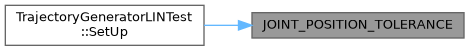## ◆ JOINT_VELOCITY_TOLERANCE()

 const std::string JOINT_VELOCITY_TOLERANCE ( "joint_velocity_tolerance" )
Here is the caller graph for this function: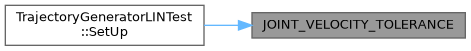## ◆ main()

 int main ( int argc, char ** argv )

Definition at line 455 of file unittest_trajectory_generator_lin.cpp.

## ◆ OTHER_TOLERANCE()

 const std::string OTHER_TOLERANCE ( "other_tolerance" )
Here is the caller graph for this function: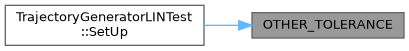## ◆ PARAM_PLANNING_GROUP_NAME()

 const std::string PARAM_PLANNING_GROUP_NAME ( "planning_group" )
Here is the caller graph for this function: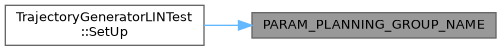## ◆ POSE_TRANSFORM_MATRIX_NORM_TOLERANCE()

 const std::string POSE_TRANSFORM_MATRIX_NORM_TOLERANCE ( "pose_norm_tolerance" )
Here is the caller graph for this function: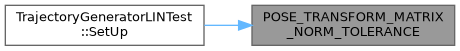## ◆ RANDOM_TEST_TRIAL_NUM()

 const std::string RANDOM_TEST_TRIAL_NUM ( "random_trial_number" )
Here is the caller graph for this function: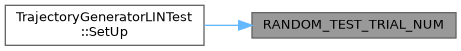## ◆ ROTATION_AXIS_NORM_TOLERANCE()

 const std::string ROTATION_AXIS_NORM_TOLERANCE ( "rot_axis_norm_tolerance" )
Here is the caller graph for this function: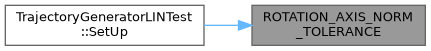Here is the caller graph for this function: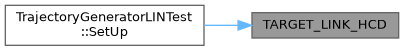## ◆ TEST_DATA_FILE_NAME()

 const std::string TEST_DATA_FILE_NAME ( "testdata_file_name" )
Here is the caller graph for this function:## ◆ TEST_F() [1/12]

 TEST_F ( TrajectoryGeneratorLINTest , cartesianSpaceGoal )

test the lin planner with Cartesian goal

Definition at line 269 of file unittest_trajectory_generator_lin.cpp.

## ◆ TEST_F() [2/12]

 TEST_F ( TrajectoryGeneratorLINTest , cartesianTrapezoidProfile )

test the trapezoid shape of the planning trajectory in Cartesian space

The test checks translational path for a trapezoid velocity profile. Due to the way the acceleration is calculated 1 or 2 intermediate points occur that are neither acceleration, constant or deceleration.

+++++++++++++++++++++++

• plan LIN trajectory + +++++++++++++++++++++++

Definition at line 291 of file unittest_trajectory_generator_lin.cpp.

Here is the call graph for this function:## ◆ TEST_F() [3/12]

 TEST_F ( TrajectoryGeneratorLINTest , cartGoalFrameIdBothConstraints )

Set a frame id in goal constraint with cartesian goal on both position and orientation constraints.

Definition at line 438 of file unittest_trajectory_generator_lin.cpp.

## ◆ TEST_F() [4/12]

 TEST_F ( TrajectoryGeneratorLINTest , cartGoalIncompleteStartState )

test invalid motion plan request with incomplete start state and cartesian goal

Definition at line 420 of file unittest_trajectory_generator_lin.cpp.

## ◆ TEST_F() [5/12]

 TEST_F ( TrajectoryGeneratorLINTest , IncorrectJointNumber )

Checks that generate() function returns 'false' if called with an incorrect number of joints.

Test Sequence:

1. Call functions with incorrect number of joints.

Expected Results:

1. Function returns 'false'.

Definition at line 402 of file unittest_trajectory_generator_lin.cpp.

## ◆ TEST_F() [6/12]

 TEST_F ( TrajectoryGeneratorLINTest , jointSpaceGoal )

test the lin planner with joint space goal

Definition at line 232 of file unittest_trajectory_generator_lin.cpp.

## ◆ TEST_F() [7/12]

 TEST_F ( TrajectoryGeneratorLINTest , jointSpaceGoalNearZeroStartVelocity )

test the lin planner with joint space goal with start velocity almost zero

Definition at line 249 of file unittest_trajectory_generator_lin.cpp.

## ◆ TEST_F() [8/12]

 TEST_F ( TrajectoryGeneratorLINTest , LinPlannerDiscontinuousJointTraj )

test joint linear movement with discontinuities in joint space

This will violate joint velocity/acceleration limits.

Test Sequence:

1. Generate lin trajectory which is discontinuous in joint space.

Expected Results:

1. Function returns 'false'.

Definition at line 346 of file unittest_trajectory_generator_lin.cpp.

Here is the call graph for this function: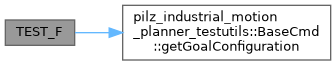## ◆ TEST_F() [9/12]

 TEST_F ( TrajectoryGeneratorLINTest , LinPlannerLimitViolation )

Check that lin planner returns 'false' if calculated lin trajectory violates velocity/acceleration or deceleration limits.

Test Sequence:

1. Call function with lin request violating velocity/acceleration or deceleration limits.

Expected Results:

1. Function returns 'false'.

Definition at line 326 of file unittest_trajectory_generator_lin.cpp.

Here is the call graph for this function:## ◆ TEST_F() [10/12]

 TEST_F ( TrajectoryGeneratorLINTest , LinStartEqualsGoal )

test joint linear movement with equal goal and start

Test Sequence:

1. Call function with lin request start = goal

Expected Results:

1. trajectory generation is successful.

Definition at line 370 of file unittest_trajectory_generator_lin.cpp.

Here is the call graph for this function: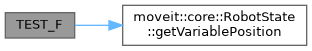## ◆ TEST_F() [11/12]

 TEST_F ( TrajectoryGeneratorLINTest , nonZeroStartVelocity )

test the lin planner with invalid motion plan request which has non zero start velocity

Definition at line 216 of file unittest_trajectory_generator_lin.cpp.

## ◆ TEST_F() [12/12]

 TEST_F ( TrajectoryGeneratorLINTest , TestExceptionErrorCodeMapping )

Checks that each derived MoveItErrorCodeException contains the correct error code.

Definition at line 189 of file unittest_trajectory_generator_lin.cpp.

## ◆ VELOCITY_SCALING_FACTOR()

 const std::string VELOCITY_SCALING_FACTOR ( "velocity_scaling_factor" )
Here is the caller graph for this function: